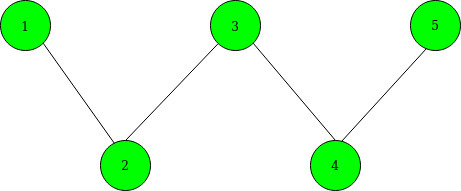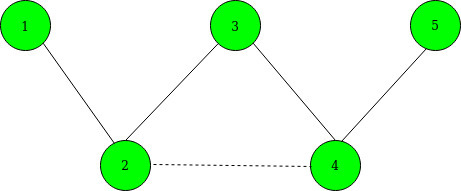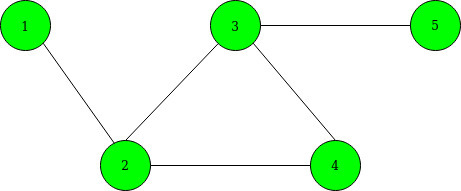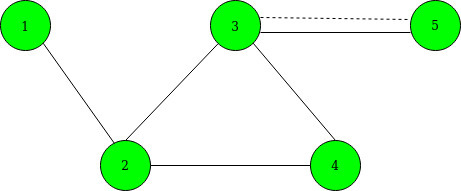Related Articles
Maximize shortest path between given vertices by adding a single edge
• Last Updated : 13 Jan, 2021

Given an undirected graph of N nodes and M vertices. You are also given a K edges as selected[]. The task to maximize the shortest path length between node 1 to node N by adding single edges between any two vertices from the given selected edges.
Note: You may add an edge between any two selected vertices who have already an edge between them.

Input: N = 5, M = 4, K = 2, selected[] = {2, 4}
Below is the given graph:Output:
Explanation:
Before adding an edge between 2 and 4, the Shortest Path becomes: 1–>2–>3–>4–>5.
After adding an edge between 2 and 4, the Shortest Path becomes 1–>2–>4–>5. Below is the graph after adding edges. denoted by the dashed line.Input: N = 5 M = 5 K = 3 selected[] = {1, 3, 5}
Below is the given graph:Output:
Explanation:
We can add an edge between 3 and 5 as they have already an edge between them. so, the shortest path becomes 1–>2–>3–>5. Below is the graph after adding edges. denoted by the dashed line.Approach: The idea is to use Breadth-First Search to find the distance from vertices 1 and N to each selected vertex. For selected vertex i, let xi denote the distance to node 1, and yi denote the distance to node N. Below are the steps:

1. Maintain a 2D matrix(say dist[]) having 2 rows and N columns.
2. In the first row, Maintain the shortest distance between node 1 and other vertices in the graph using BFS Traversal.
3. In the second row, Maintain the shortest distance between node N and the other vertices of the graph using BFS Traversal.
4. Now, choose two selected vertices a and b from selected[] to minimize the value of min(xa + yb, ya + xb). For this do the following:
• Create a vector of pairs and store the value of (xi – yi) with their respective selected node.
• Sort the above vector of pairs.
• Initialize best to 0 and max to -INF.
• Now traverse the above vector of pairs and for each selected node(say a) update the value of best to maximum of (best, max + dist[a]) and update max to maximum of (max, dist[a]).
5. After the above operations the maximum of (dist[N-1] and best + 1) given the minimum shortest path.

Below is the implementation of the above approach:

## C++

 `// C++ program for the above approach``#include ``using` `namespace` `std;``const` `int` `INF = 1e9 + 7;``int` `N, M;` `// To store graph as adjacency list``vector<``int``> edges;` `// To store the shortest path``int` `dist;` `// Function that performs BFS Traversal``void` `bfs(``int``* dist, ``int` `s)``{``    ``int` `q;` `    ``// Fill initially each distance as INF``    ``fill(dist, dist + N, INF);``    ``int` `qh = 0, qt = 0;``    ``q[qh++] = s;``    ``dist[s] = 0;` `    ``// Perform BFS``    ``while` `(qt < qh) {` `        ``int` `x = q[qt++];` `        ``// Traverse the current edges``        ``for` `(``int` `y : edges[x]) {``            ``if` `(dist[y] == INF) {` `                ``// Update the distance``                ``dist[y] = dist[x] + 1;` `                ``// Insert in queue``                ``q[qh++] = y;``            ``}``        ``}``    ``}``}` `// Function that maximizes the shortest``// path between source and destination``// vertex by adding a single edge between``// given selected nodes``void` `shortestPathCost(``int` `selected[], ``int` `K)``{``    ``vector > data;` `    ``// To update the shortest distance``    ``// between node 1 to other vertices``    ``bfs(dist, 0);` `    ``// To update the shortest distance``    ``// between node N to other vertices``    ``bfs(dist, N - 1);` `    ``for` `(``int` `i = 0; i < K; i++) {` `        ``// Store the values x[i] - y[i]``        ``data.emplace_back(dist[selected[i]]``                              ``- dist[selected[i]],``                          ``selected[i]);``    ``}` `    ``// Sort all the vectors of pairs``    ``sort(data.begin(), data.end());``    ``int` `best = 0;``    ``int` `MAX = -INF;` `    ``// Traverse data[]``    ``for` `(``auto` `it : data) {``        ``int` `a = it.second;``        ``best = max(best,``                   ``MAX + dist[a]);` `        ``// Maximize x[a] - y[b]``        ``MAX= max(MAX, dist[a]);``    ``}` `    ``// Print minimum cost``    ``printf``(``"%d\n"``, min(dist[N - 1], best + 1));``}` `// Driver Code``int` `main()``{``    ``// Given nodes and edges``    ``N = 5, M = 4;``    ``int` `K = 2;``    ``int` `selected[] = { 1, 3 };` `    ``// Sort the selected nodes``    ``sort(selected, selected + K);` `    ``// Given edges``    ``edges.push_back(1);``    ``edges.push_back(0);``    ``edges.push_back(2);``    ``edges.push_back(1);``    ``edges.push_back(3);``    ``edges.push_back(2);``    ``edges.push_back(4);``    ``edges.push_back(3);` `    ``// Function Call``    ``shortestPathCost(selected, K);``    ``return` `0;``}`

## Java

 `// Java program for the above approach``import` `java.util.*;``import` `java.lang.*;` `class` `GFG{``    ` `static` `int` `INF = (``int``)1e9 + ``7``;``static` `int` `N, M;``  ` `// To store graph as adjacency list``static` `ArrayList> edges;``  ` `// To store the shortest path``static` `int``[][] dist = ``new` `int``[``2``][``200000``];``  ` `// Function that performs BFS Traversal``static` `void` `bfs(``int``[] dist, ``int` `s)``{``    ``int``[] q = ``new` `int``[``200000``];``  ` `    ``// Fill initially each distance as INF``    ``Arrays.fill(dist, INF);``    ` `    ``int` `qh = ``0``, qt = ``0``;``    ``q[qh++] = s;``    ``dist[s] = ``0``;``  ` `    ``// Perform BFS``    ``while` `(qt < qh)``    ``{``        ``int` `x = q[qt++];``  ` `        ``// Traverse the current edges``        ``for``(Integer y : edges.get(x))``        ``{``            ``if` `(dist[y] == INF)``            ``{``                ` `                ``// Update the distance``                ``dist[y] = dist[x] + ``1``;``  ` `                ``// Insert in queue``                ``q[qh++] = y;``            ``}``        ``}``    ``}``}``  ` `// Function that maximizes the shortest``// path between source and destination``// vertex by adding a single edge between``// given selected nodes``static` `void` `shortestPathCost(``int` `selected[], ``int` `K)``{``    ``ArrayList<``int``[]> data = ``new` `ArrayList<>();``  ` `    ``// To update the shortest distance``    ``// between node 1 to other vertices``    ``bfs(dist[``0``], ``0``);``  ` `    ``// To update the shortest distance``    ``// between node N to other vertices``    ``bfs(dist[``1``], N - ``1``);``  ` `    ``for``(``int` `i = ``0``; i < K; i++)``    ``{``        ` `        ``// Store the values x[i] - y[i]``        ``data.add(``new` `int``[]{dist[``0``][selected[i]] -``                           ``dist[``1``][selected[i]],``                                   ``selected[i]});``    ``}``  ` `    ``// Sort all the vectors of pairs``    ``Collections.sort(data, (a, b) -> a[``0``] - b[``0``]);``    ``int` `best = ``0``;``    ``int` `MAX = -INF;``  ` `    ``// Traverse data[]``    ``for``(``int``[] it : data)``    ``{``        ``int` `a = it[``1``];``        ``best = Math.max(best,``                        ``MAX + dist[``1``][a]);``  ` `        ``// Maximize x[a] - y[b]``        ``MAX = Math.max(MAX, dist[``0``][a]);``    ``}``    ` `    ``// Print minimum cost``    ``System.out.println(Math.min(dist[``0``][N - ``1``],``                                     ``best + ``1``));``}` `// Driver code``public` `static` `void` `main (String[] args)``{``    ` `    ``// Given nodes and edges``    ``N = ``5``; M = ``4``;``    ``int` `K = ``2``;``    ``int` `selected[] = { ``1``, ``3` `};``    ` `    ``// Sort the selected nodes``    ``Arrays.sort(selected);``    ` `    ``edges = ``new` `ArrayList<>();``    ` `    ``for``(``int` `i = ``0``; i < ``200005``; i++)``        ``edges.add(``new` `ArrayList());``    ` `    ``// Given edges``    ``edges.get(``0``).add(``1``);``    ``edges.get(``1``).add(``0``);``    ``edges.get(``1``).add(``2``);``    ``edges.get(``2``).add(``1``);``    ``edges.get(``2``).add(``3``);``    ``edges.get(``3``).add(``2``);``    ``edges.get(``3``).add(``4``);``    ``edges.get(``4``).add(``3``);``    ` `    ``// Function Call``    ``shortestPathCost(selected, K);``}``}` `// This code is contributed by offbeat`

## Python3

 `# Python3 program for the above approach` `# Function that performs BFS Traversal``def` `bfs(x, s):``    ``global` `edges, dist``    ``q ``=` `[``0` `for` `i ``in` `range``(``200000``)]` `    ``# Fill initially each distance as INF``    ``# fill(dist, dist + N, INF)``    ``qh, qt ``=` `0``, ``0``    ``q[qh] ``=` `s``    ``qh ``+``=` `1``    ``dist[x][s] ``=` `0` `    ``# Perform BFS``    ``while` `(qt < qh):``        ``xx ``=` `q[qt]``        ``qt ``+``=` `1` `        ``# Traverse the current edges``        ``for` `y ``in` `edges[xx]:``            ``if` `(dist[x][y] ``=``=` `10``*``*``18``):` `                ``# Update the distance``                ``dist[x][y] ``=` `dist[x][xx] ``+` `1` `                ``# Insert in queue``                ``q[qh] ``=` `y``                ``qh ``+``=` `1` `# Function that maximizes the shortest``# path between source and destination``# vertex by adding a single edge between``# given selected nodes``def` `shortestPathCost(selected, K):``    ``global` `dist, edges``    ``data ``=` `[]` `    ``# To update the shortest distance``    ``# between node 1 to other vertices``    ``bfs(``0``, ``0``)` `    ``# To update the shortest distance``    ``# between node N to other vertices``    ``bfs(``1``, N ``-` `1``)``    ``for` `i ``in` `range``(K):` `        ``# Store the values x[i] - y[i]``        ``data.append([dist[``0``][selected[i]]``-` `dist[``1``][selected[i]], selected[i]])` `    ``# Sort all the vectors of pairs``    ``data ``=` `sorted``(data)``    ``best ``=` `0``    ``MAX` `=` `-``10``*``*``18` `    ``# Traverse data[]``    ``for` `it ``in` `data:``        ``a ``=` `it[``1``]``        ``best ``=` `max``(best,``MAX` `+` `dist[``1``][a])` `        ``# Maximize x[a] - y[b]``        ``MAX``=` `max``(``MAX``, dist[``0``][a])` `    ``# Prminimum cost``    ``print``(``min``(dist[``0``][N ``-` `1``], best ``+` `1``))` `# Driver Code``if` `__name__ ``=``=` `'__main__'``:` `    ``# Given nodes and edges``    ``edges ``=` `[[] ``for` `i ``in` `range``(``5``)]``    ``dist ``=` `[[``10``*``*``18` `for` `i ``in` `range``(``1000005``)] ``for` `i ``in` `range``(``2``)]``    ``N,M ``=` `5``, ``4``    ``K ``=` `2``    ``selected ``=` `[``1``, ``3``]` `    ``# Sort the selected nodes``    ``selected ``=` `sorted``(selected)` `    ``# Given edges``    ``edges[``0``].append(``1``)``    ``edges[``1``].append(``0``)``    ``edges[``1``].append(``2``)``    ``edges[``2``].append(``1``)``    ``edges[``2``].append(``3``)``    ``edges[``3``].append(``2``)``    ``edges[``3``].append(``4``)``    ``edges[``4``].append(``3``)` `    ``# Function Call``    ``shortestPathCost(selected, K)` `    ``# This code is contributed by mohit kumar 29`
Output:
`3`

Time Complexity: O(N*log N + M)
Auxiliary Space: O(N)

My Personal Notes arrow_drop_up